C481 B581 Computer Graphics
Dana Vrajitoru

### Geometrical modeling

• Scalars, points, vectors
• Curves
• Surfaces
• Solid objects
Scalars, Points, Vectors
Scalar - a real number, a quantity.

Point - P(x, y, z), a location in space. 0-dimensional object in the 3D space. In OpenGL a point can be drawn with
glBegin(GL_POINTS);
glVertexf(x, y, z); // as many as we need
glEnd();

Vector - V(x, y, z), a combination of a direction and a magnitude. A vector does not have a fixed position in space. The usual representation for some physical quantities like velocity, force.

Parametric Curves

Def. P(x(s), y(s), z(s)), a < s < b. Usually a=0, b=1.
Notation: P(x(s), y(s), z(s)) = P(s).
1-dimensional object in the 3D space depending on one parameter s.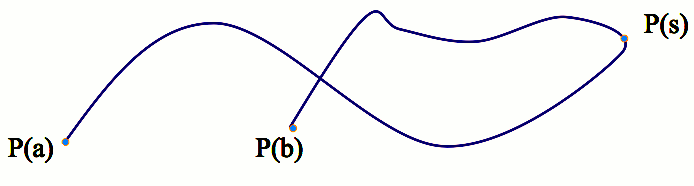Examples:

 Line segment from (x1, y1, z1) to (x2, y2, z2) x(s) = (1-s) x1 + s x2 y(s) = (1-s) y1 + s y2 z(s) = (1-s) z1 + s z2 0 < s < 1 Circle in the Oxy plane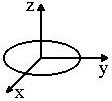x 2  +  y2  =  r2 z=0 x(s) = r cos s y(s) = r sin s z(s) = 0 0 < s < 2 pi Spiral in the Oxy plane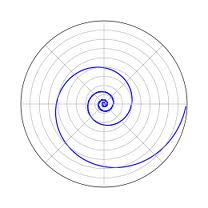General equation: x2 + y2 = (r(s))2, z=0 x(s) = c s cos s y(s) = c s sin s z(s) = 0 0 < s < 5 pi Helix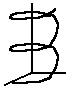x 2  +  z2  =  r2 0 < y < 3 r x(s) = r cos s y(s) = r s / (2 pi) z(s) = r sin s 0 < s < 6 pix(s) = 0 y(s) = (2 - cos s) cos s z(s) = 2 sin s 0 < s < 2 pi

Parametric Surfaces

Def. P(x(s, t), y(s, t), z(s, t)), a < s < b, c < t < d.
2-dimensional objects in the 3D space depending on two parameters s and t.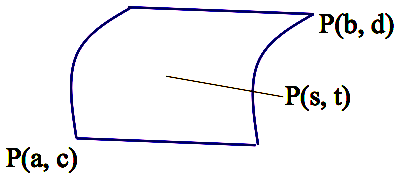Examples:

 Triangle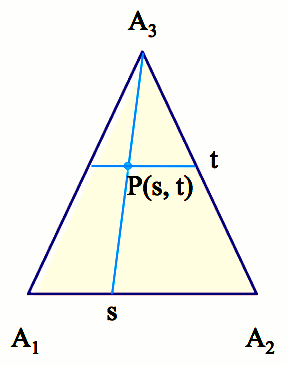A1(x1, y1, z1) , A2(x2, y2, z2), A3(x3, y3, z3) x(s, t) = (1-t)((1-s) x1 + s x2) + t x3 y(s, t) = (1-t)((1-s) y1 + s y2) + t y3 z(s, t) = (1-t)((1-s) z1 + s z2) + t z3 0 < s < 1, 0 < t < 1 Ellipsoid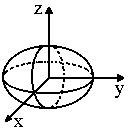(x/a) 2 + (y/b)2 + (z/c)2 =  1 x(s) = a cos s cos t y(s) = b sin s cos t z(s) = c sin t 0 < s < 2 pi, 0 < t < 2 pi Cylinder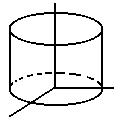x 2  +  y2  =  r2 0 < z < a x(s, t) = r cos s y(s, t) = r sin s z(s, t) = t 0 < s < 2 pi, 0 < t < a Cone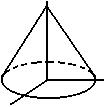x 2  +  y2  =  (1 - z)2 0 < z < 1 x(s, t) = (1 - t) cos s y(s, t) = (1 - t) sin s z(s, t) = t 0 < s < 2 pi, 0 < t < 1 Rotation surface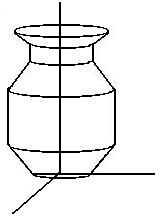Given a planar curve  (x1, y1) construct the rotation  surface around 0y: x 2  +  z2 =  x12 y = y1 (x1(s), y1(s)) x(s, t) = x1(s) cos t y(s, t) = y1(s) z(s, t) = x1(s) sin t

Solid objects

Def. P(x(s, t, u), y(s, t, u), z(s, t, u)), a < s < b, c < t < d, e < u < f;
3-dimensional objects in the 3D space depending on three parameters s, t, and u;
In CG they are represented by the bounding surface which is closed.
Sometimes we need to visualize the whole volume, like for medical images.

### Discretization

• The 3D equivalent of scan converting from the 2D.
• For a curve: starting from the general continuous parameterization, representing the curve as a sequence of (small) line segments.
• For a surface: starting with the general continuous parameterization, representing the surface as a collection of polygons, usually triangles or quadrilaterals.
• A volume is usually discretized as a collection of units of volume (voxels) represented as points, spheres, cubes, etc.

Discretizing curves.
Consider the curve x(s), y(s), z(s), a < s < b.
We want to draw the curve as a number of  nlines line segments.

step = (b - a) / nlines;
for (i=0; i<nlines; i++) {
s1 = a + i * step;
s2 = a + (i+1) * step;
Line3D(x(s1), y(s1), z(s1),
x(s2), y(s2), z(s2));
}

In OpenGL: represent the curve as a line strip.
step = (b - a) / nlines;
glBegin(GL_LINE_STRIP);
for (i=0; i<=nlines; i++) {
s = a + i * step;
glVertex3f((x(s), y(s), z(s));
}
glEnd();

Discretizing surfaces.
Consider the surface x(s, t), y(s, t), z(s, t), a < s < b, c < t < d.
We want to discretize it using spoints along s and tpoints along t.

point(s, t) : x(s, t), y(s, t), z(s, t).

sstep = (b - a) / spoints;
tstep = (d - c) / tpoints;
for (i=0; i<spoints; i++) {
s1 = a + i * sstep;
s2 = a + (i+1) * sstep;
for (j=0; j<tpoints; j++) {
t1 = c + j * tstep;
t2 = c + (j+1) * tstep;

// First model
Polygon(point(s1, t1), point(s1, t2),
point(s2, t2), point(s2, t1),
point(s1, t1));

// Second model
Triangle(point(s1, t1), point(s1, t2), point(s2, t1));
Triangle(point(s1, t2), point(s2, t1), point(s2, t2));
}

If the triangle or the polygon is drawn as a collection of lines, we have wireframe models.

In OpenGL: represent each band in the surface for given s1 and s2 as a triangle strip.
sstep = (b - a) / spoints;
tstep = (d - c) / tpoints;
for (i=0; i<spoints; i++) {
s1 = a + i * sstep;
s2 = a + (i+1) * sstep;
glBegin(GL_TSTRIP);
for (j=0; j<=tpoints; j++) {
t = c + j * tstep;
glVertex3f(x(s1,t), y(s1,t), z(s1,t));
glVertex3f(x(s2,t), y(s2,t), z(s2,t));
}
glEnd();
}